Thread: Eric Dollard View Single Postt-rexSenior Member Join Date: Aug 2011 Location: In the bushes Posts: 422
Law of Electro-Magnetic Induction, Four

(1) The following analysis begins with chapter three, Law of Electro-Magnetic Induction by C.P. Steinmetz, 1916. This chapter opens with the definition of one volt of electro-motive force. One volt of E.M.F. is developed by the movement of 100 million lines of magnetic induction. This movement develops its E.M.F. via the metallic-dielectric geometry bounding the magnetic induction. Hereby the relation is given as,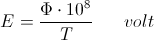And,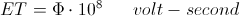The volt-second defines the unit of magnetic charge, just as the ampere-second defines the unit of electric charge. Hence, and E.M.F. of one volt, over a duration of one second, produces or consumes one unit of magnetic induction, this induction consisting of 100 million lines of magnetic flux. One flux line, or tube of induction, can be taken as a discrete entity, and of a distinct dimensional size, this a numerical constant. In other words a quantum size of magnetic induction defines all lines of induction as being the same size. It is then that this line of induction is a constituent of the Planck, a quantum dimensional relation consisting in part of a quantum of magnetic induction. The factor of ten to the eighth power partially defines the size of the Planck, in defining a distinct line of magnetic induction. Here is a partial answer to the old question of how big is a Planck?

(2) The volt-second, or magnetic charge is the conjugate of the ampere-second, or dielectric charge. Magnetic charge is in weber, dielectric charge is in coulomb, this dimensionally given as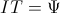In common practice the unit of dielectric charge is the ampere-hour, and defines the quantity of electricity into, or out of, an electric accumulator (storage battery).

Considering the ampere-second it is when the displacement current, I, in amperes, passes thru an impedance, Z, in ohm, that energy is exchanged with the dielectric field of induction. In this passage the displacement current, I, in amperes is transformed into a magneto-motive force, i, also in amperes. This i relates to the metallic, and the displacement I relates to the dielectric. When this impedance, Z, consists of a resistance, R, in ohm, the displacement current gives rise to an electronic current, i, this also in amperes, the energy in the dielectric is dissipated at the rate,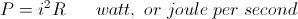(3) The concept of the accumulation of charge is normally not considered when dealing with a magnetic field and the energy it contains. This magnetic charge is analogous to the dielectric charge. Magnetic charge is given as the volt-second, this a volt of E.M.F. When this volt of E.M.F. is impressed upon an admittance, Y, in siemens, that energy is exchanged with the magnetic field of induction. Hereby the E.M.F., E, in volt, is transformed into an electro-static potential, e, also in volt. When this electro-static potential is impressed upon a conductance, G, in siemens, this conductance dissipates the energy stored in the magnetic field of induction at the rate,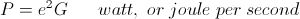It was shown in the Four Quadrant Theory, E.P. Dollard, that dielectric discharges give rise to strong currents, whereas magnetic discharges give rise to high E.M.F.s. This was shown in recent experiments with the 60KV, 3000KVA, power line transformer. The magnetic charge drawn from only a 12 volt car battery can have destructive consequences if discharged rapidly by an open circuit, hence the flashover on the bushing safety gap.

(4) Electro-motive force is the medium by which energy is supplied to, or demanded of, the magnetic field developing this E.M.F. When this E.M.F. is made to be an electro-static potential, then energy can be exchanged. Part of this energy is stored, thru a displacement current,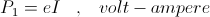or it is dissipated,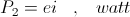or combined, for an A.C. wave,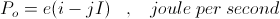Gives the total electrical activity of the magnetic field of induction in the exchange of its energy to an external form. In this relation the E.M.F., E, is made equivalent to electro-static potential, e, this via a parallel connection of the magnetic inductance to the external system. Conversely, for the dielectric field, the displacement current, I, is made equivalent to M.M.F., or electronic current, i, this via a series connection of the dielectric capacity to the external system. Hence the Law of Electro-Magnetic Induction is entirely analogous to the Law of Dielectric Induction, and the understanding of one can be derived from the other.

BK DE N6KPH
__________________
SUPPORT ERIC DOLLARD'S WORK AT EPD LABORATORIES, INC.

Purchase Eric Dollard's Books & Videos - 70% of the sale goes to Eric and EPD Laboratories: Eric Dollard Books & Videos
Donate by Paypal: Donate to EPD Laboratories